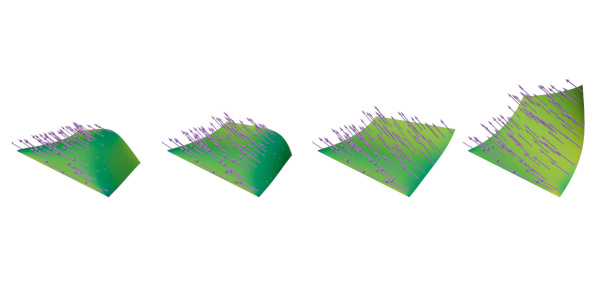## Error estimates for elastic flowsThin elastic structures lead to a variety of interesting phenomena and applications. Often, two energy stable bending states are used to create a quasistationary switching device or to generate mechanical locomotion. For all of these technical developments large deformations of thin elastic objects are important. Their mathematical modeling leads to constrained bending energies in which the constraint captures the resistance to shearing and stretching effects of thin elastic plates or the inextensibility and twist behavior of an elastic rod with small cross section. Different finite element discretizations of such energies have recently been devised. By following ideas for the numerical approximation of harmonic maps, which describe director fields with values in given manifolds, practical and convergent numerical methods were obtained. In parallel research the derivation of error estimates for classes of geometric evolution problems was addressed, which include the Willmore, the mean curvature, and the harmonic map heat flow. Crucial in those developments was the identification of an equivalent formulation of the evolution problems as a nonlinear parabolic system that includes equations for geometric quantities such as the normal field. In this project we aim at bringing the developments together to obtain error estimates for finite element discretizations of time stepping schemes for gradient flows of various bending energies. The motivation for this is twofold: first, this justifies the numerical simulation of natural, strongly damped relaxation dynamics, and second, it leads to error estimates for the approximation of stationary states obtained via certain evolution processes. In addition, this serves as a step towards simulating general dynamical processes that capture inertial effects, e.g., vibrating elastic rods. Other applications include the determination of stationary states of magnetic elastic films and rods.

### Publications

 Sören Bartels, Balázs Kovács, and Zhangxian Wang, Error analysis for the numerical approximation of the harmonic map heat flow with nodal constraints, arXiv e-prints, 2208.08267 [math.NA], 2022. [bibtex]